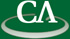## Sparse polynomial powers using heaps.

### Roman Pearce, Center for Experimental and Constructive Mathematics, Simon Fraser University

July 11th at 3:45pm in K9509.

```We discuss powering of sparse polynomials, which is
surprisingly complicated.  In most computer algebra
systems, the best algorithm for expanding f^k is to
repeatedly multiply by f.  Repeated squaring, as in
f^4 = (f^2)^2, is much worse for sparse polynomials.
In this talk we describe a new method which expands
f^k for about the cost of multiplying f^(k-1) and f.
It has much better  complexity and lower  space for
powering  dense  polynomials  which  makes it  more
attractive  for use  as a general  purpose routine.
We show how to parallelize the method,  and compare
its performance on a series  of  benchmark problems
to other methods and the Magma,  Maple and Singular
computer algebra systems.

This is joint work with Michael Monagan.
```